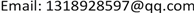Landsat OLI数据，土地利用分类，分层分类，植被覆盖度, Landsat OLI Data Land Use Classification Hierarchical Classification Vegetation Coverage1. 引言

2. 实验方法2.1. 基于决策树的分层分类方法

2.2. 精度评价

K = ( p 1 − p 2 ) / ( 1 − p 2 ) (1)

p 2 = ( a 1 ∗ b 1 + a 2 ∗ b 2 + ⋯ + a 0 ∗ b 0 ) / n 2 (2)

2.3. 植被覆盖度反演方法

f c = ( S − S soil ) / ( S veg − S soil ) (3)

f c = ( NDVI − NDVI soil ) / ( NDVI veg − NDVI soil ) (4)

3. 实验过程

3.1. 分类过程

3.2. 精度评价

K = N ∑ i = 1 r   x i i − ∑ i = 1 r ( x i + x + i ) N 2 − ∑ i = 1 r ( x i + x + i ) (5)

3.3. 植被覆盖度反演

4. 结束语